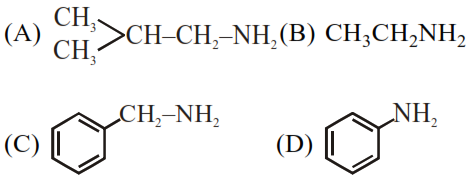# The total number of amines among the followingQuestion:

The total number of amines among the following which can be synthesized by Gabriel synthesis isSolution:

Gabriel phthalimide synthesis is used to prepare $1^{\circ}$ aliphatic/alicyclic amine in common.

Hence amine which can synthesised by Gabriel phthalimide synthesis method is :

(A) $\mathrm{Me}_{2} \mathrm{CH}-\mathrm{CH}_{2}-\mathrm{NH}_{2}$ (B) $\mathrm{CH}_{3} \mathrm{CH}_{2} \mathrm{NH}_{2}$

(C) $\mathrm{Ph}-\mathrm{CH}_{2}-\mathrm{NH}_{2}$